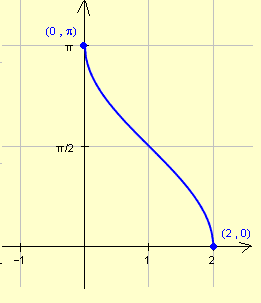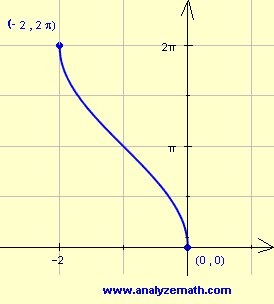# Graphing arccos(x) functions

A step by step tutorial on graphing and sketching arccos(x) functions and also the domain and range of these functions and other properties are discussed.

## Graph, Domain and Range of arccos(x)

In what follows, arccos(x) is the inverse function of f(x) = cos(x) for 0 ≤ x ≤ π.
The domain of y = arccos(x) is the range of f(x) = cos(x) for 0 ≤ x ≤ π and is given by the interval [-1 , 1]. The range of arccos(x) is the domain of f which is given by the interval [0 , π].
The graph, domain and range of both f(x) = cos(x) for 0 ≤ x ≤ π and arccos(x) are shown below.A table of values of arccos(x) can made as follows:
 x -1 0 1 y = arccos(x) π π/2 0

Note that there are 3 key points that may be used to graph arccos(x). These points are: (-1,π) , (0,π/2) and (1,0).

### Example 1

Find the domain and range of y = arccos(x - 1) and graph it.

Solution to Example 1
The graph of y = arccos(x - 1) will be that of arccos(x) shifted 1 unit to the right. The domain is found by stating that -1 ≤ x - 1 ≤ 1. Solve the double inequality to find the domain:
0 ≤ x ≤ 2
The 3 key points of arccos(x) can also be used in this situation as follows:

 x - 1 -1 0 1 y = arccos(x-1) π π/2 0 x 0 1 2

The value of x is calculated from the value of x - 1. For example when x - 1 = -1, solve for x to find x = 0 and so on.
The domain is given by the interval [0,2] and the range is given by the interval [0,π]
The three points will now be used to graph y = arccos(x - 1).### Example 2

Find the domain and range of y = 2 arccos(x + 1) and graph it.

Solution to Example 2 We use the 3 key points in the table as follows, then find the value 2 arccos(x + 1) and x.

 x + 1 -1 0 1 arccos(x+1) π π/2 0 y = 2 arccos(x+1) 2π π 0 x -2 -1 0

domain = [-2,0] , range = [0 , 2 π]The graph is that of arccos(x) shifted one unit to the left and stretched vertically by a factor of 2.

### Example 3

Find the domain and range of y = - arccos(x - 1) and graph it.

Solution to Example 3 We use the 3 key points in the table as follows, then find the value - arccos(x - 1) and x.

 x - 1 -1 0 1 arccos(x-1) π π/2 0 y = - arccos(x-1) -π -π/2 0 x 0 1 2

domain = [0 , 2] , range = [- π , 0]The graph is that of arccos(x) shifted one unit to the right and reflected on the x axis.

## More References and Links to Graphing

Graphing Functions